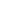#Formulas and Functions MSO-1004 is a Course

## Formulas and Functions MSO-1004

Self-paced

### Full course description

Course Description

Formulas and functions enable you to perform calculations on a worksheet. Excel can perform simple calculations, such as summing a column of numbers, or very complex calculations such as calculating financial, statistical or engineering information.

By the end of this module, you will be able to

• Define formula and function

• List the mathematical operators used in Excel

• Explain how references to cells or ranges are used in formulas and functions

• Use the Sum and Average functions

• Use the AutoSum command

• Understand how to copy formulas and functions

• Explain relative and absolute cell references

• Understand how to move formulas and functions

• Describe methods for troubleshooting formulas and functions

Estimated completion time (hours): 1.7